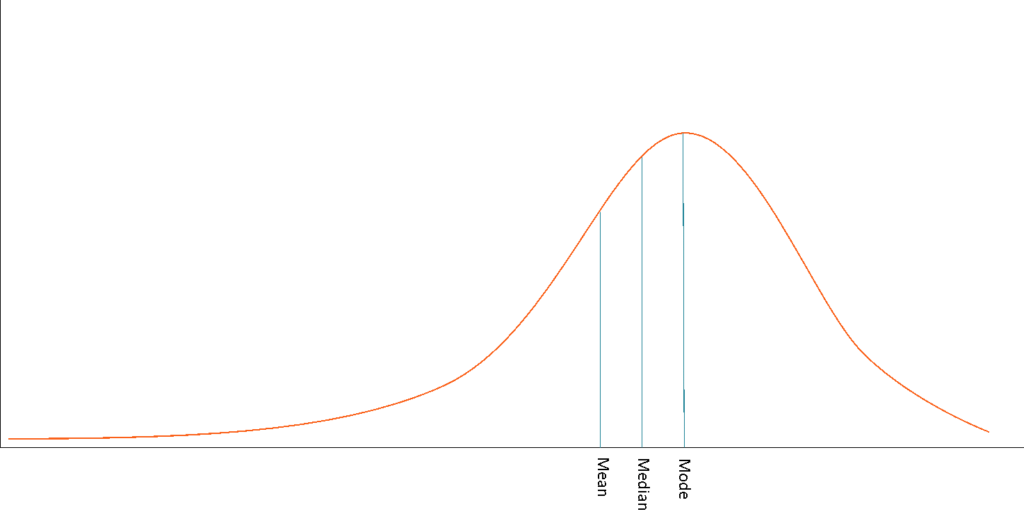# Negatively Skewed Distribution

A type of distribution in which more values are concentrated on the right side (tail) of the distribution graph

## What is a Negatively Skewed Distribution?

In statistics, a negatively skewed (also known as left-skewed) distribution is a type of distribution in which more values are concentrated on the right side (tail) of the distribution graph while the left tail of the distribution graph is longer.While normal distribution is the most commonly encountered type of distribution, examples of the negatively skewed distributions are also widespread in real life. A negatively skewed distribution is a direct opposite of a positively skewed distribution.

### Central Tendency Measures in Negatively Skewed Distributions

Unlike normally distributed data where all measures of central tendency (mean, median, and mode) equal each other, in a negatively skewed data, the measures are dispersed. The general relationship between the central tendency measures in a negatively skewed distribution may be expressed using the following inequality:

##### Mode  >  Median  > Mode

Another important note about the measures of central tendency in negatively skewed distributions is that the arithmetic mean is generally located on the left from the peak of the distribution. Although the rules mentioned previously are considered to be the general rules for negatively skewed distributions, you may encounter many exceptions in real life that violate the rules.

The significant negative skewness of a distribution may not be suitable for thorough statistical analysis. The high skewness of the data may lead to misleading results from the statistical tests. Due to such a reason, negatively skewed data goes through the transformation process to make it close to the normal distribution. The statistical tests are usually run only when the transformation of the data is complete.

### Negatively Skewed Distribution in Finance

In finance, the concept of skewness is utilized in the analysis of the distribution of the returns on investments. Although many finance theories and models assume that the returns of securities follow a normal distribution, in reality, the returns are usually skewed.

The negative skewness of the distribution indicates that an investor may expect frequent small gains and few large losses. In reality, many trading strategies employed by traders are based on negatively skewed distributions.

Despite the fact that strategies based on negative skewness may provide stable profits, an investor or a trader should be aware that there is still a probability of large losses. Thus, it is imperative to properly assess the risks of the trading strategies and include the skewness of the returns in the assessment.